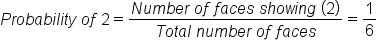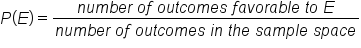# Theoretical Probability/A Priori Method

Author: Sophia Tutorial
##### Description:

Calculate theoretical probability given a certain situation.

(more)

Sophia’s self-paced online courses are a great way to save time and money as you earn credits eligible for transfer to many different colleges and universities.*

No credit card required

37 Sophia partners guarantee credit transfer.

299 Institutions have accepted or given pre-approval for credit transfer.

* The American Council on Education's College Credit Recommendation Service (ACE Credit®) has evaluated and recommended college credit for 32 of Sophia’s online courses. Many different colleges and universities consider ACE CREDIT recommendations in determining the applicability to their course and degree programs.

Tutorial
what's covered
This tutorial will discuss the Theoretical Probability Model (also known as "a priori method"). Our discussion breaks down as follows:

1. Theoretical Probability Model
2. Unlikely Equal Outcomes

## 1. Theoretical Probability Model

A fairly common way to thinking about probability is to enumerate all the outcomes and assign them each an equal probability.

### IN CONTEXT

When you roll a die, there are six results since they are numbered: 1, 2, 3, 4, 5, or 6. Those are the outcomes.

Suppose you wanted to determine the probability of rolling a 2. Only one of those faces shows a 2, and there are six total faces, so the probability of you rolling a 2 is, therefore, one out of six.The understanding here is that the faces--1, 2, 3, 4, 5, and 6--are equally likely. This is the key! There are other events whose probabilities can be calculated using this method.

Other probabilities calculated using this method are based on the key idea that outcomes are equally likely. Anytime you have outcomes that are equally likely, the theoretical probability method can be used, such as:

• A coin flip
• Drawing a card from a deck (the deck must be adequately shuffled)
• Selecting marbles from a jar (all marbles must be equal size so you have an equal probability of picking them out). They should also be well mixed.

The basic formula for theoretical probability is:

formula
Theoretical Probabilityterm to know

Theoretical Probability
The method of assigning probability to events based on the assumption that all events are equally likely. The probability of an event is the equally likely ways an event can occur divided by the number of possible outcomes.

## 2. Unlikely Equal Outcomes

The theoretical probability model does not work if the outcomes aren't equally likely.

EXAMPLE

You could use this reasoning to say, for example, you could get home safely from work in your car, or you could get in a horrible car crash. Either is a possible outcome. If you assign those equal likelihoods, then the probability of you getting into a car crash is one half, because there are only two outcomes. You either make it home safe, or you get in a crash.

However, we know from experience that the outcome of not having an accident is so much more likely than the outcome of having an accident. Therefore, equal probabilities are not assigned in this case because experience shows that those two outcomes are not equally likely.

did you know
The theoretical probability model is also called "priori method," which means it is known prior to, or beforehand.

summary
The Theoretical Probability Model (or a priori method) states that when you have equally likely outcomes in some kind of chance experiment, the probabilities that you can determine for events is calculated by taking the number of outcomes in the event divided by the total number of possible outcomes. The caveat of this model, however, is that this only works when the events are equally likely, or the outcomes are equally likely. It does not work when the outcomes are not equally likely.

Good luck!

Source: Adapted from Sophia tutorial by Jonathan Osters.

Terms to Know
Theoretical Probability

The method of assigning probability to events based on the assumption that all events are equally likely. The probability of an event is the equally likely ways an event can occur divided by the number of possible outcomes.

Formulas to Know
Theoretical ProbabilityRating